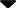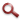Site WebYou are here:

## Student Project FiguresFigure 2 Figure 2.  Shannon's Diversity Index calculated per plate. p = the proportion that phyla makes of the total count of species within phyla present. Natural log, is the natural log of the proportion, which is then multiplied by p. H = the sum of all p x natural log results. H'max is H/ natural log of 10 (the number of phyla) * -1 (to get a positive number). The H max indicates the final SDI score, so this plate has a diversity index of 0.658. Figure 2